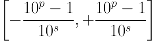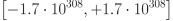## Numeric Data Types

Exasol supports approximate and exact numerical data types. The difference when using the approximate data types arises from the possible rounding differences, which can occur due to the type of storage and the way computations are performed. Information losses of this nature do not occur when using exact numeric types because of storage type.

Exasol Type (ANSI type) Range (min.,max.) Precision Note
Exact numeric type
DECIMAL(p,s)Precision, Scale: (p,s)

s ≤ p≤ 36

p ≥ 1 and s ≥ 0

Approximate numeric type
DOUBLE PRECISION~15 digits About 15 digits can be stored, independent of the scale. The special element NaN is interpreted as NULL, the element Infinity is not supported